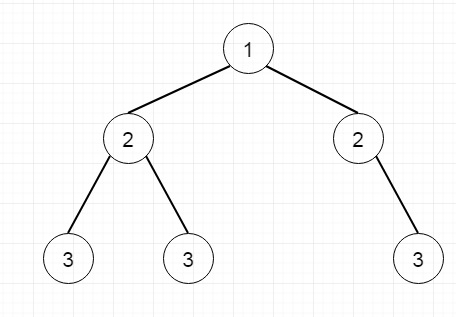# Maximum Depth of Binary Tree in Python

Suppose we have one binary tree. We have to find the maximum depth of that tree. The maximum depth of a tree is the maximum number of nodes that are traversed to reach the leaf from the root using the longest path. Suppose the tree is like below. The depth will be 3 here.To solve this, we will follow these steps.

• Here we will use the recursive approach. The method is solve(root, depth = 0)
• if the root is empty, then return depth
• otherwise return max of solve(left, depth + 1) and solve(left, depth + 1)

Let us see the following implementation to get a better understanding −

## Example

Live Demo

class TreeNode:
def __init__(self, data, left = None, right = None):
self.data = data
self.left = left
self.right = right
def insert(temp,data):
que = []
que.append(temp)
while (len(que)):
temp = que
que.pop(0)
if (not temp.left):
if data is not None:
temp.left = TreeNode(data)
else:
temp.left = TreeNode(0)
break
else:
que.append(temp.left)
if (not temp.right):
if data is not None:
temp.right = TreeNode(data)
else:
temp.right = TreeNode(0)
break
else:
que.append(temp.right)
def make_tree(elements):
Tree = TreeNode(elements)
for element in elements[1:]:
insert(Tree, element)
return Tree
class Solution(object):
def maxDepth(self, root):
"""
:type root: TreeNode
:rtype: int
"""
return self.solve(root)
def solve(self,root,depth = 0):
if root == None:
return depth
return max(self.solve(root.left,depth+1),self.solve(root.right,depth+1))
tree1 = make_tree([1,2,2,3,4,None,3])
ob1 = Solution()
print(ob1.maxDepth(tree1))

## Input

tree1 = make_tree([1,2,2,3,4,None,3])

## Output

3